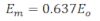# Mean Value Or Average Value Of AC

The mean value or average value of AC over a complete cycle is zero. So we do not consider the average value of AC over one cycle. We therefore take the average value of AC over a half cycle only I.e. from t=0 to t=T/2
The average value of AC over a half cycle is that value of steady current that would send same amount of charge in same interval of time that would be sent by the DC in same time.
To calculate the mean value of ac, Let us consider an ac given by: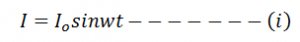The small amount of charge sent by an ac in small time dt is: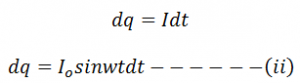Total charge carried by ac in half cycle is obtained by integrating equation ii from t = 0 to t = T/2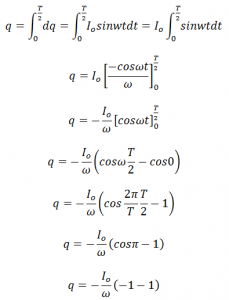If Im be the average ac then charge carried by it in half cycle is:

Now from equation iii and iv we get,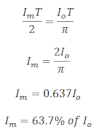Similarly the average value of ac over negative half cycle (t = T/2 to t = T) is: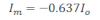Therefore the average ac over a complete cycle is zero.

Similarly the average value of alternating emf over a half cycle is: